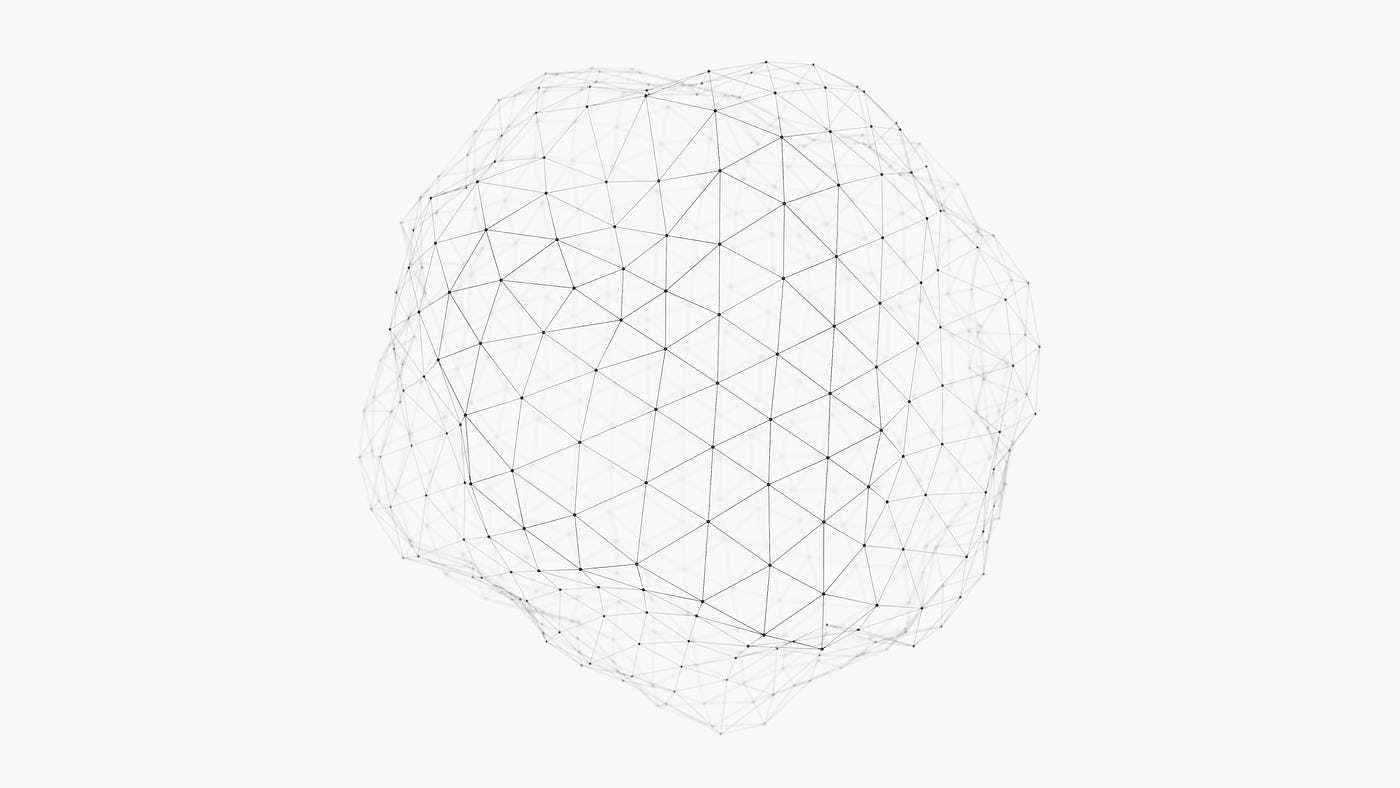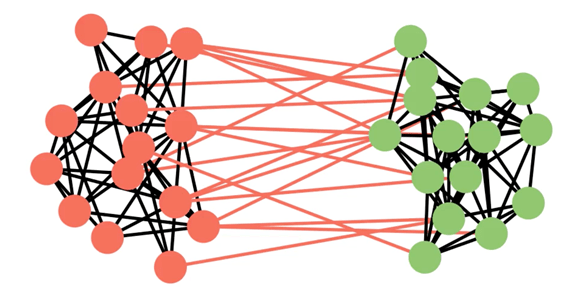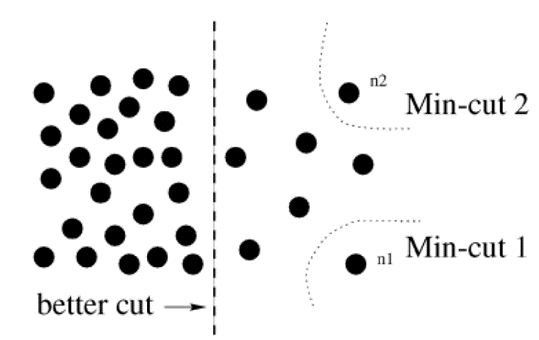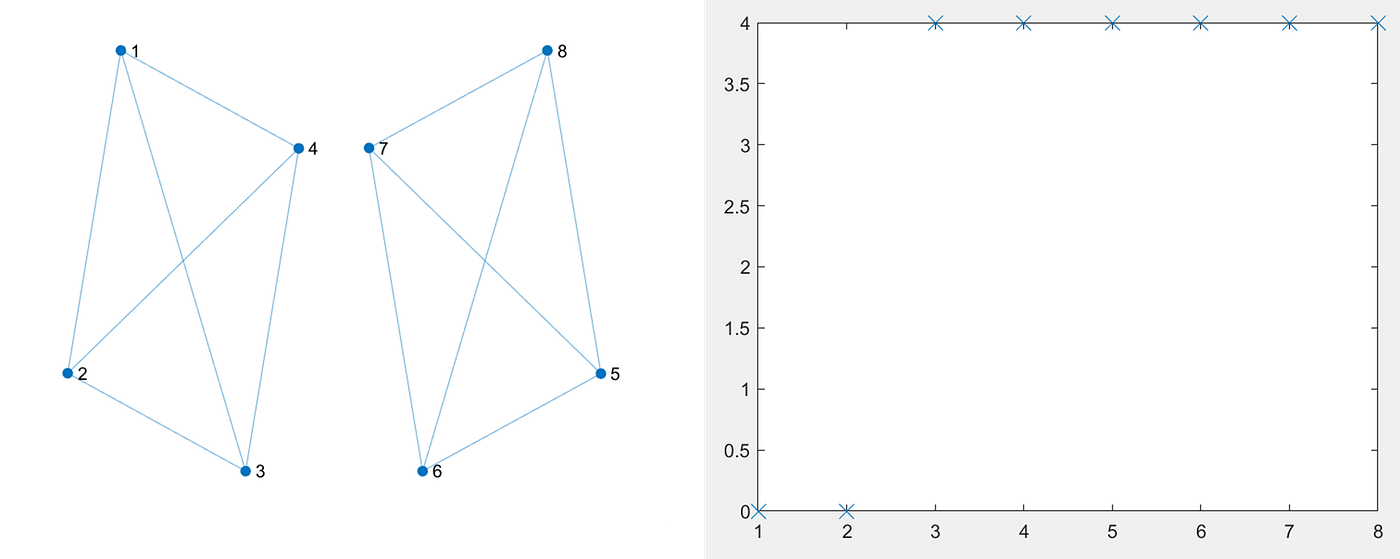# An Introduction to Graph Partitioning Algorithms and Community Detection | by Shanon Hong | Aug, 2022Photo by D koi on Unsplash

Graph partitioning has been a long-lasting problem and has a wide range of applications. This post shares the methodology for graph partitioning with both theoretical explanations and practical implementations of some popular graph partitioning algorithms with python codes.

## Clarification

“Clustering” can be confusing under different contexts. In this article, clustering means node clustering, i.e. partitioning the graphs into clusters (or communities). We use graph partitioning, (node) clustering, and community detection interchangeably. In other words, we do not consider overlapping communities anywhere in this article. (Do note that a wider definition of community detection can include overlapping communities)

Graph partitioning is usually an unsupervised process, where we define the desired quality measure, i.e. clustering evaluation metrics, then we employ some algorithms to find the best partitioning solution based on the defined evaluation metrics. In the remaining content, we will first go through the two most popularly used evaluation metrics. Then we introduce two methods that can effectively find the (approximated) solution for each evaluation metric.

As clustering is usually an unsupervised task, it is important to have a quality measure or objective function to evaluate the clustering solutions. Clustering in other domains usually uses different distance-based functions over the data points to measure the quality of clustering. However, as graphs are non-Euclidean data and the distance of data point is far less as important as the connections in the graph, we need to have different measurements for clustering in a graph. In other words, the quality measure needs to be defined over the graph connectivity.

## Cut and Normalized-Cut

In general, a portion of nodes and edges are recognized as one community (or one cluster) if they are densely connected to each other, otherwise considered as different communities. Therefore, it is natural to cluster the graph such that the nodes have maximum edges within one cluster and minimum edges across different clusters. The sum of weights of edges across different clusters is defined as the ‘cut’ of the graph. In other words, ‘cut’ is the total weight of edges removed to completely disconnect the graph into separate sub-graphs.Simple Illustration of Cut. Image by Author

Mathematically, the ‘cut’ of a graph G into two disjoint sets A and B can be computed as:

To find the best way of clustering the graph G, the problem is equivalent to finding the minimum value of “cut”, i.e. min-cut. However, it is also not hard to see that one possible degenerated solution of min-cut is cutting off a small number of nodes from the entire graph, resulting in trivial clusters with only a few edges removed. One degenerated solution is illustrated inAn example of trivial solutions by min-cut. Figure from .

To overcome this problem,  proposed a method to normalize the cuts over the measurement of the volume of edges inside each cluster, defined as the association (Assoc).

Normalized-cut (n-cut) effectively penalizes the degenerated solutions in min-cut, making it a robust and popular clustering measure in many applications including image segmentation and graph community detection.

## Modularity

Graph modularity was introduced in  as a quality function to evaluate the compactness of communities. The modularity Q is defined as:

where 2m is the volume of edges, A is the graph adjacency matrix, k_i and k_j are the degrees of node i and node j, s_i and s_j are the community indicator.

Interpretation of graph modularity:

A_ij is the actual number of edges between every two nodes, since the graph adjacency matrix is what defines the graph connectivity. And the expression (k_i*k_j)/2m gives the expected number of edges (assuming edges are placed at random) between every two nodes, or in other words, the probability of an edge existing between node i and node j (if edges are placed at random).

Therefore, modularity can be interpreted as the difference between the actual number of edges and the expected number of edges (assuming edges are placed at random) for every pair of nodes in one community (or one cluster).

Modularity takes the value between [-0.5, 1] and is at maximum when the community is disconnected from the remaining of the graph and is at minimum when the community contains fully disconnected nodes.

We can easily test a graph partitioning solution using the two evaluation metrics mentioned earlier. However, finding the(best) graph partitioning solution is known to be NP-complete. In other words, there is no known efficient algorithm that can solve this problem more efficiently than a Brute Force one. In layman’s terms, the only possible way to guarantee the best solution is by trying every possible combination…Due to the size of graphs, it almost exhibits exhaustively test (e.g. Brute Force) for all combinations of graph clustering solutions. Therefore, over the years, different efficient algorithms have been proposed for finding the approximated solutions for graph clustering.

## Spectral Clustering

It is well known that the min-cut problem can be solved efficiently by the Ford-Fulkerson algorithm. However, when the size balancing condition is imposed (i.e. normalized-cut), this problem becomes NP-Complete. Spectral clustering is a method to approximate the solutions for normalized-cut through eigenvalue/eigenvector decomposition over the normalized graph Laplacian.

• Obtain graph Laplacian by: L=D-A
• Perform eigen decomposition of graph Laplacian. Lv=λv
• Project graph to eigenvectors corresponding to the k smallest eigenvalues, with each column of projected eigenvectors a eigen-feature for each node
• perform k-mean clustering on eigen features

The eigenvalues indicate the connectivity of a graph. The eigenvalues of graph Laplacian give us insight into the variation when we traverse down a graph. The smallest eigenvalue is always zero. The number of zeroed eigenvalues indicates the number of the connected components in the graph. This can be seen from the examples below.Graph with two connected components. Image by AuthorGraph with one connected component. Image by Author

If you look at the eigenvalues closely, you would notice there is a sudden change in the spectrum. I usually call this the ‘eigen-gap’ (though I noticed there are different definitions for this). The gap indicates the number of naturally existing clusters in the graph. This can be observed in the examples below:Graph with three natural clusters. Image by AuthorGraph with two natural clusters. Image by Author

Notice the place of the occurrence of the ‘gap’ changes from ‘3’ to ‘2’, exactly corresponding to the number of clusters in the graph.

This observation gives us the intuition for using the eigenvalues to find the number of clusters in the graph, especially when we don’t know the actual or the expected number of clusters in our data (which is very often is real-world use cases).

Next, all we need to do is to project the eigenvectors to each node of the graph. In the example below, we have previously identified three clusters in the eigenvalue plot. Therefore, we take only the first three eigenvectors corresponding to the smallest three eigenvalues. Note that each eigenvector contains exactly N numbers, where N is the number of nodes in the graph. We project the first three eigenvectors to each node, with each column as the eigen features. A K-means clustering (or any other clustering method on data points you prefer) is performed to find the clusters based on the eigenfeatures. The colors in the following example indicate the identified clusters. Note that only the first three rows (i.e. first three eigenvectors) are used in the computation. You can do a quick verification by looking at the distances of eigenfeatures in each cluster. Note that I purposely make {1,5,6} in one cluster in the graph to show that the node index does not matter.Illustration of clustering on eigenvectors. Image by Author

Alright, that’s the maths behind spectral clustering. In real cases, you don’t really need to go through all these complex implementations unless you are a researcher or you want to fully understand what you are doing. A simpler way to employ spectral clustering is using the implementation in sklearn Library. An example is shown in the code block below:

`import networkx as nxfrom sklearn.cluster import SpectralClusteringfrom sklearn.metrics.cluster import normalized_mutual_info_scoreimport numpy as np# Here, we create a stochastic block model with 4 clusters for evaluationsizes = [150, 150, 150, 150]        probs = [[0.20, 0.05, 0.02, 0.03], [0.05, 0.30, 0.07, 0.02],                 [0.02, 0.07, 0.30, 0.05], [0.03, 0.02, 0.05, 0.50]]G = nx.stochastic_block_model(sizes, probs, seed=0)adj = nx.adjacency_matrix(G)n_clusters = 4node_labels = [G.nodes[n]['block'] for n in np.sort(G.nodes)]spectral_clusters = SpectralClustering(n_clusters=n_clusters, assign_labels="discretize", affinity='precomputed').fit_predict(adj)# Get the resultnmi = normalized_mutual_info_score(spectral_clusters, node_labels)print("nmi:", nmi)`

Limitations:

1. Eigen-decomposition of a large matrix is computationally very expensive. This exhibits spectral clustering to be applied on large graphs.
2. Spectral clustering is only an approximation for the optimal clustering solutions.

## Louvain Clustering

Louvain’s method  is a fast algorithm for graph modularity optimization. It optimizes the modularity of a graph in a 2-phase iterative process. In phase 1, it starts by assigning each node in the graph a separate community. After that, for each node i, the algorithm evaluates the change in the modularity of the graph when :

1. node i is removed from its original community
2. node i is inserted into the community of its neighboring node j

Phase 1 repeats until there is no increase in modularity and the local maximum is hit.

During phase 2, a new graph is created by replacing all nodes in the same community are merged into one single node representing the community. Edges within the community are replaced by self-loop to the node, and edges outside the community are replaced by weighted edges to other nodes. Once the new graph is created, it repeats phase 1 on the new graph.

We will use the implementation in sknetwork to test Louvain’s method. An example is shown in the code block below:

`import networkx as nxfrom sknetwork.clustering import Louvainfrom sklearn.metrics.cluster import normalized_mutual_info_scoreimport numpy as np# Here, we create a stochastic block model with 4 clusters for evaluationsizes = [150, 150, 150, 150]        probs = [[0.20, 0.05, 0.02, 0.03], [0.05, 0.30, 0.07, 0.02],                 [0.02, 0.07, 0.30, 0.05], [0.03, 0.02, 0.05, 0.50]]G = nx.stochastic_block_model(sizes, probs, seed=0)adj = nx.adjacency_matrix(G)n_clusters = 4node_labels = [G.nodes[n]['block'] for n in np.sort(G.nodes)]louvain = Louvain()    clusters = louvain.fit_transform(adj)# Get the resultnmi = normalized_mutual_info_score(clusters, node_labels)print("nmi:", nmi)`

In this article, we briefly introduced graph partitioning, two evaluation metrics for graph partitioning, and two types of algorithms that optimize n-cut and graph modularity respectively. These algorithms are early methods that can be traced back to the 2000s but are still widely used for many graph partitioning applications due to their great efficiency and feasibility.

However, graphs in recent applications usually contain rich information in the node features. Therefore, although powerful and efficient, these methods are becoming less and less applicable to modern graph applications. The limitation of these methods is that they only partition graphs based on graph connectivity but they do not consider any information in node features. Though some works have been done to encode part of node features into the edge weight and perform partitioning on a weighted graph, the amount of information that can be represented by edge weight is still limited. There are recent methods using Graph Neural Network for graph partitioning which can jointly consider graph connectivity and node features to detect communities in the graph.

 J. Shi and J. Malik, “Normalized Cuts and Image Segmentation”, IEEE TRANSACTIONS ON PATTERN ANALYSIS AND MACHINE INTELLIGENCE, vol. 22, no. 8, pp. 888–905, 2000.

 M. E. J. Newman, “Modularity and community structure in networks”, Phy. Rev., 2006

 Blondel, Vincent D., Guillaume, Jean-Loup, Lambiotte, Renaud, Lefebvre, Etienne, “Fast unfolding of communities in large networks”, Journal of Statistical Mechanics, 2008